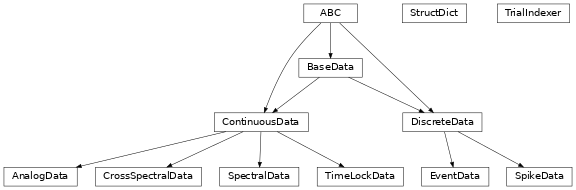# Complete API#

Reference to the complete Syncopy API

## syncopy Package#

syncopy.startup_print_once(message, force=False)[source]#

Print message once: do not spam message n times during all n worker imports.

### Functions#

 `definetrial`(obj[, trialdefinition, pre, ...]) (Re-)define trials of a Syncopy data object `selectdata`(data[, trials, channel, ...]) Create a new Syncopy object from a selection `show`(data[, squeeze]) Show (partial) contents of Syncopy object `copy`(spydata) Create a copy of the entire Syncopy object data on disk `redefinetrial`(data_obj[, trials, minlength, ...]) This function allows you to adjust the time axis of your data, i.e. to change from stimulus-locked to response-locked. `concat`(spy_obj1, spy_obj2[, dim]) Concatenate two Syncopy data objects trial-by-trial along dim axis. `load_ft_raw`(filename[, list_only, ...]) Imports raw time-series data from Field Trip into potentially multiple `AnalogData` objects, one for each structure found within the MAT-file. `load_tdt`(data_path[, start_code, end_code, ...]) Imports TDT time series data and meta-information into a single `AnalogData` object. `load_nwb`(filename[, memuse, container, ...]) Read contents of NWB files `cleanup`([older_than, interactive, ...]) Delete old files in temporary Syncopy folder Clear Syncopy objects from memory `load`(filename[, tag, dataclass, checksum, ...]) Load Syncopy data object(s) from disk `save`(out[, container, tag, filename, overwrite]) Save Syncopy data object to disk Convert raw spy.AnalogData (single-trial data) to an MNE Python RawArray. Convert MNE python mne.io.RawArray to spy.AnalogData (single-trial data). `tldata_to_mne_epochs`(tldata) Convert Syncopy timelocked data to MNE Python mne.EpochsArray. Convert MNE EpochsArray to time-locked Syncopy AnalogData instance. Parse input arguments of obj and return dictionary `freqanalysis`(data[, method, output, ...]) Perform (time-)frequency analysis of Syncopy `AnalogData` objects `connectivityanalysis`(data[, method, ...]) Perform connectivity analysis of Syncopy `SpectralData` OR directly `AnalogData` objects `spike_psth`(data[, binsize, output, latency, ...]) Peristimulus time histogram `timelockanalysis`(data[, latency, ...]) Average, variance and covariance for `AnalogData` objects across trials. `mean`(spy_data, dim[, keeptrials, parallel, ...]) Calculates the average along arbitrary dimensions of a Syncopy data object `spy_data`. `std`(spy_data, dim[, keeptrials, parallel, ...]) Calculates the standard deviation along arbitrary dimensions of a Syncopy data object `spy_data`. `var`(spy_data, dim[, keeptrials, parallel, ...]) Calculates the variance along arbitrary dimensions of a Syncopy data object `spy_data`. `median`(spy_data, dim[, keeptrials, ...]) Calculates the median along arbitrary dimensions of a Syncopy data object `spy_data`. `itc`(spec_data[, select]) Calculates the inter trial coherence for a SpectralData spec_data object, the input spectrum needs to be complex. `singlepanelplot`(data, **show_kwargs) Plot Syncopy data in a single panel `multipanelplot`(data, **show_kwargs) Plot Syncopy data in multiple panels `preprocessing`(data[, filter_class, ...]) Preprocessing of time continuous raw data with IIR and FIR filters `resampledata`(data[, resamplefs, method, ...]) Performs resampling or downsampling of `AnalogData` objects, representing uniformly sampled time series data.

### Classes#

 `AnalogData`([data, filename, ...]) Multi-channel, uniformly-sampled, analog (real float) data `SpectralData`([data, filename, ...]) Multi-channel, real or complex spectral data `CrossSpectralData`([data, filename, ...]) Multi-channel real or complex spectral connectivity data `TimeLockData`([data, filename, ...]) Multi-channel, uniformly-sampled, time-locked data. `SpikeData`([data, filename, trialdefinition, ...]) Spike times of multi- and/or single units `EventData`([data, filename, trialdefinition, ...]) Timestamps and integer codes of experimental events `TrialIndexer`(data_object, idx_list) `StructDict`(*args, **kwargs) Child-class of dict for emulating MATLAB structs

### Class Inheritance Diagram### syncopy.synthdata Package#

### Functions#

 `ar2_network`([AdjMat, nSamples, alphas, ...]) Simulation of a network of coupled AR(2) processes `ar2_peak_freq`(a1, a2[, samplerate]) Helper function to tune spectral peak of AR(2) process `collect_trials`(trial_func) Decorator to wrap around a single trial (nSamples x nChannels shaped np.ndarray) synthetic data function. `harmonic`(freq, samplerate[, nSamples, ...]) A harmonic with frequency freq. `linear_trend`(y_max[, nSamples, nChannels, ...]) A linear trend on all channels from 0 to y_max in nSamples. `mk_RandomAdjMat`([nChannels, conn_thresh, ...]) Create a random adjacency matrix for the network of AR(2) processes where entry `(i,j)` is the coupling strength from channel `i -> j` `phase_diffusion`(freq[, eps, samplerate, ...]) Linear (harmonic) phase evolution plus a Brownian noise term inducing phase diffusion around the deterministic phase velocity (angular frequency). `poisson_noise`([nTrials, nSpikes, nChannels, ...]) Poisson (Shot-)noise generator `red_noise`(alpha[, nSamples, nChannels, ...]) Uncoupled multi-channel AR(1) process realizations. `scalar_parser`(var[, varname, ntype, lims]) Parse scalars `signature`(obj, *[, follow_wrapped, globals, ...]) Get a signature object for the passed callable. `unwrap_cfg`(func) Decorator that unwraps cfg "structure" in metafunction call `white_noise`([nSamples, nChannels, seed, nTrials]) Plain white noise with unity standard deviation.